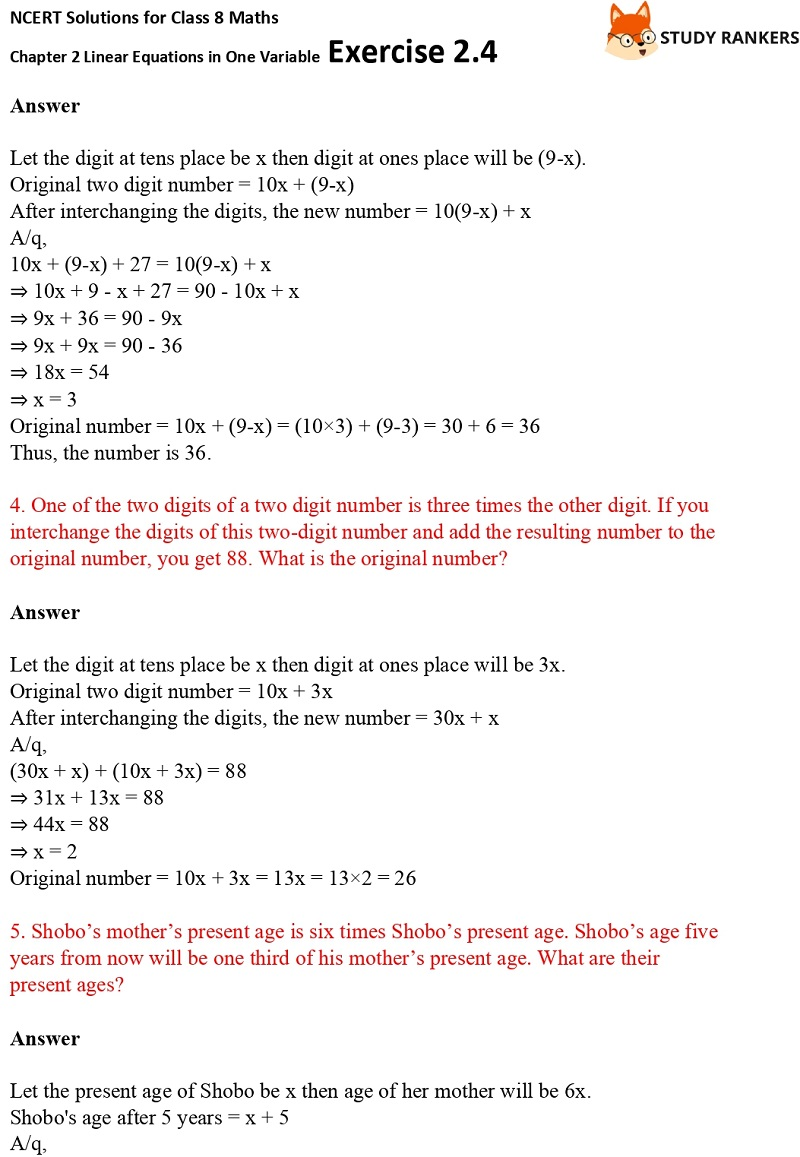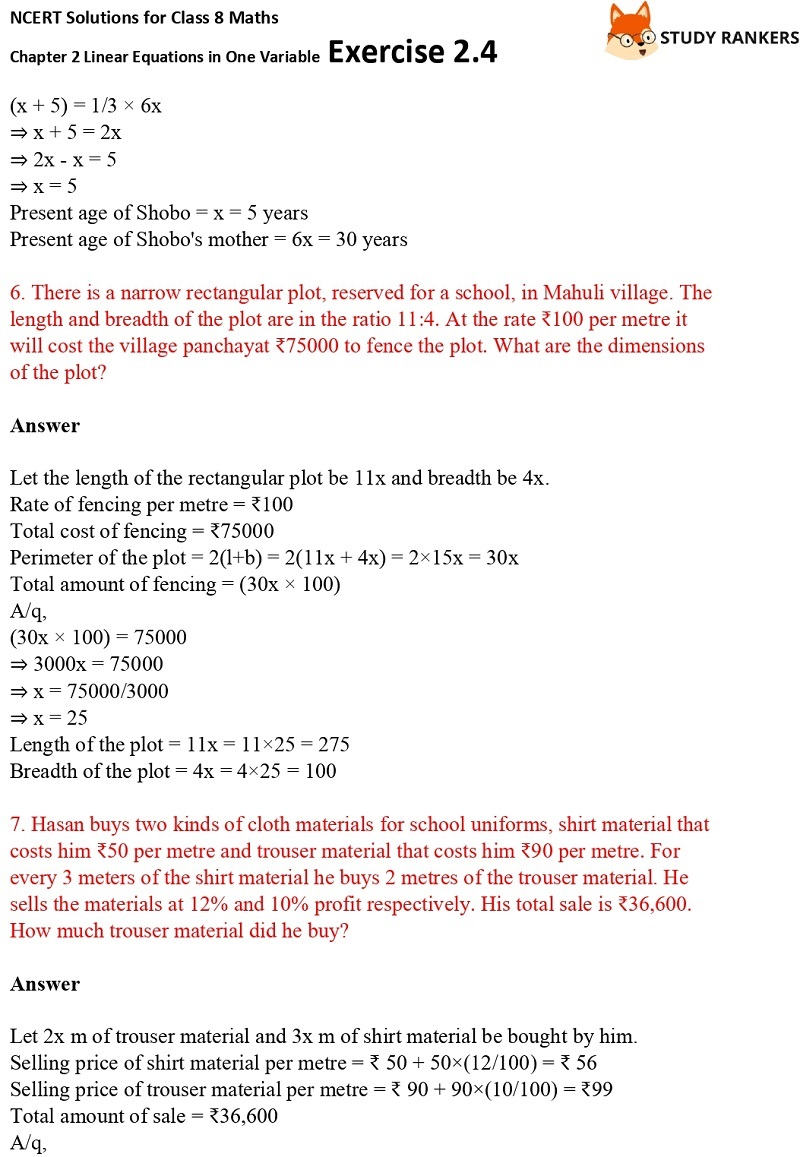>

# NCERT Solutions for Class 8 Maths Ch 2 Linear Equations in One Variable Exercise 2.4

NCERT Solutions for Class 8 Maths Ch 2 Linear Equations in One Variable Exercise 2.4 is present here which will guide you in a better way while solving any solutions. You can refer to these NCERT Solutions whenever you find any question hard to solve. You will get to now about a lot of important points given in the chapter.

Also, NCERT Solutions for Class 8 Maths provided on Studyrankers is very much helpful during the preparation of examinations. In the exercise 2.4, there are word problems which you need to put them into equations having the variable on both side.X